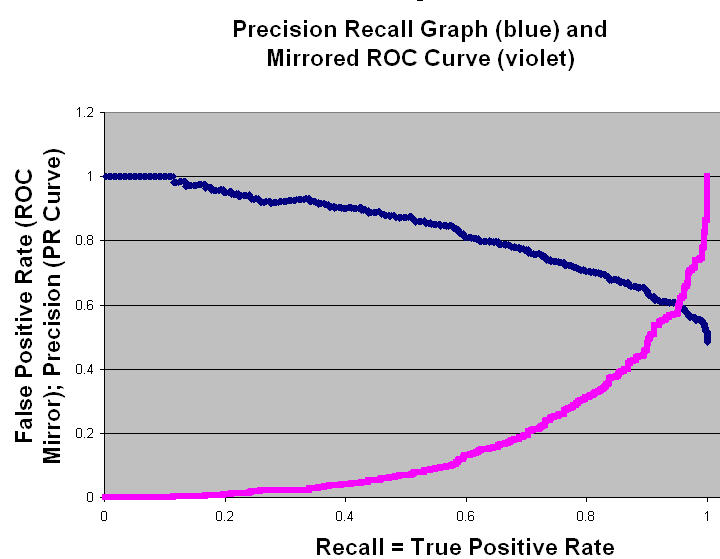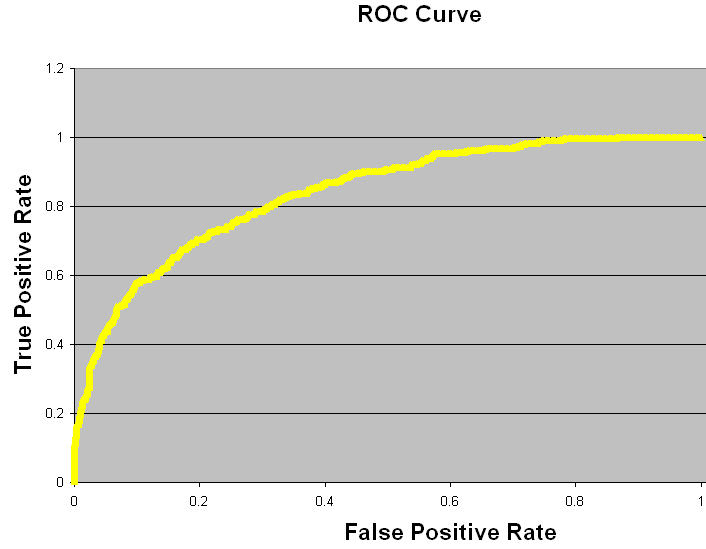There is now a better discussion of the relationship between precision/recall and ROC available in form of a book chapter.

### Precision Recall vs ROC (Receiver Operating Characteristic)

Here is a direct comparison of how a particular system is characterized by a precision recall graph vs. by an ROC curve. This is the data used to plot the two charts.

### Precision Recall Graph, Mirrored ROC Curve### ROC curve